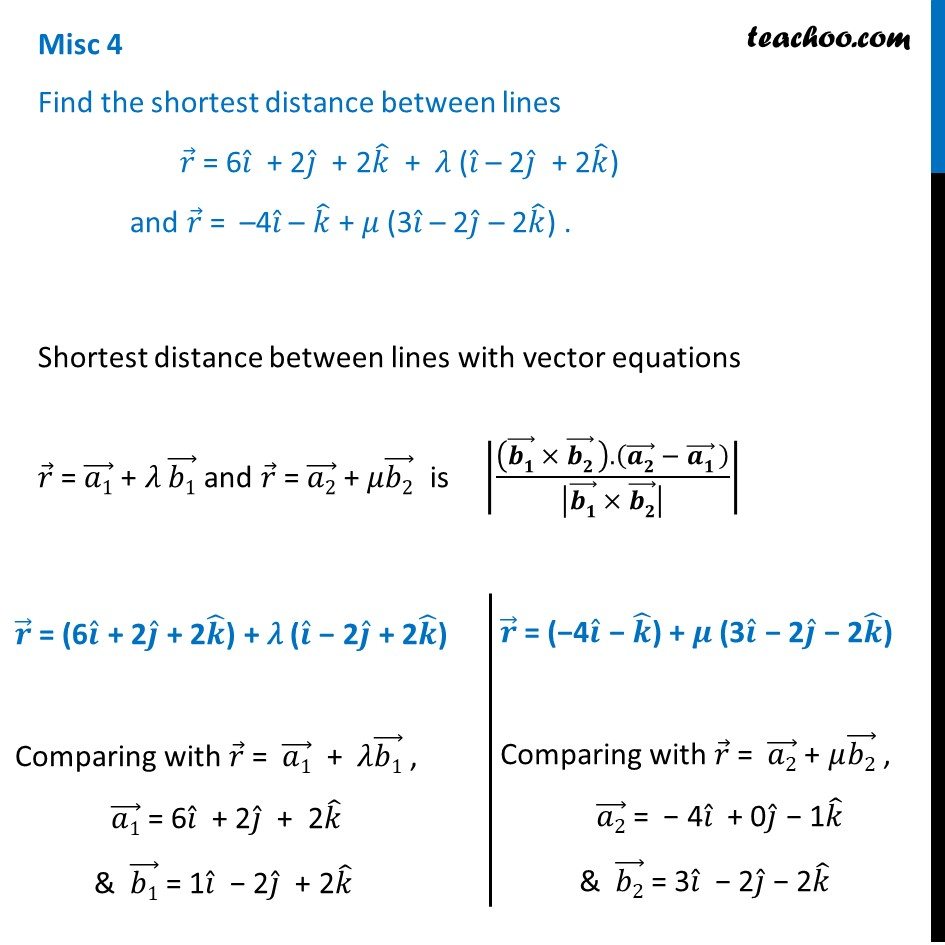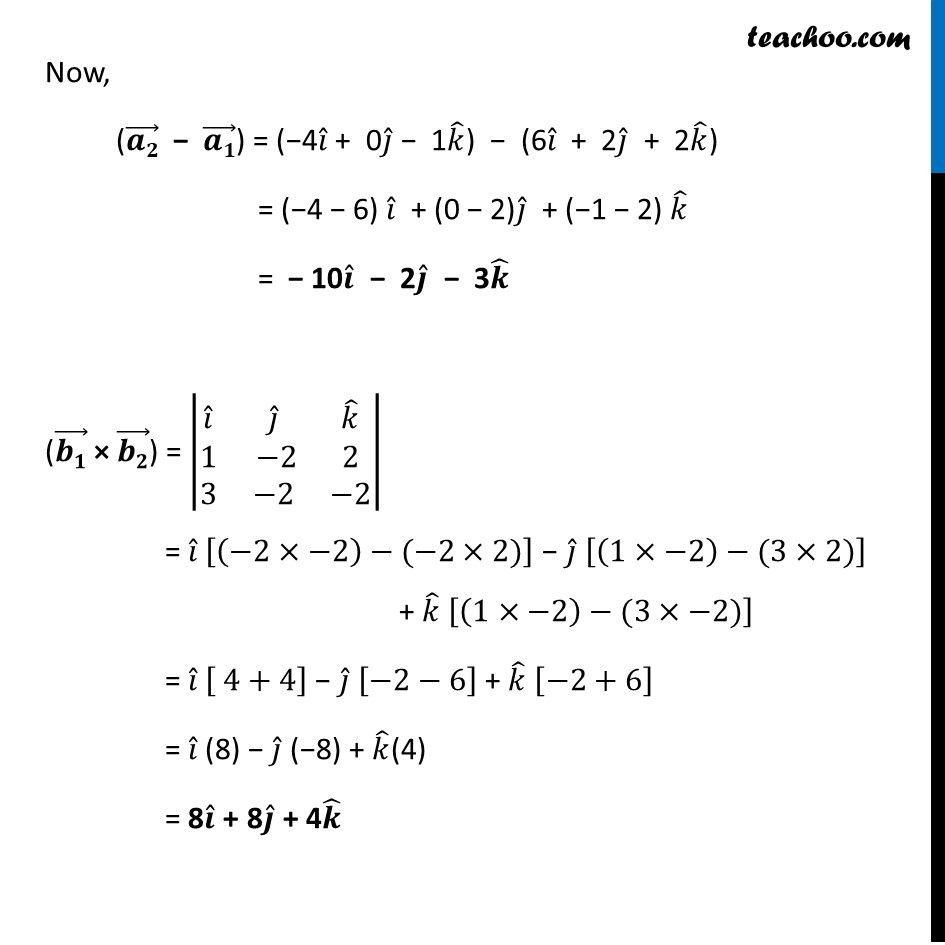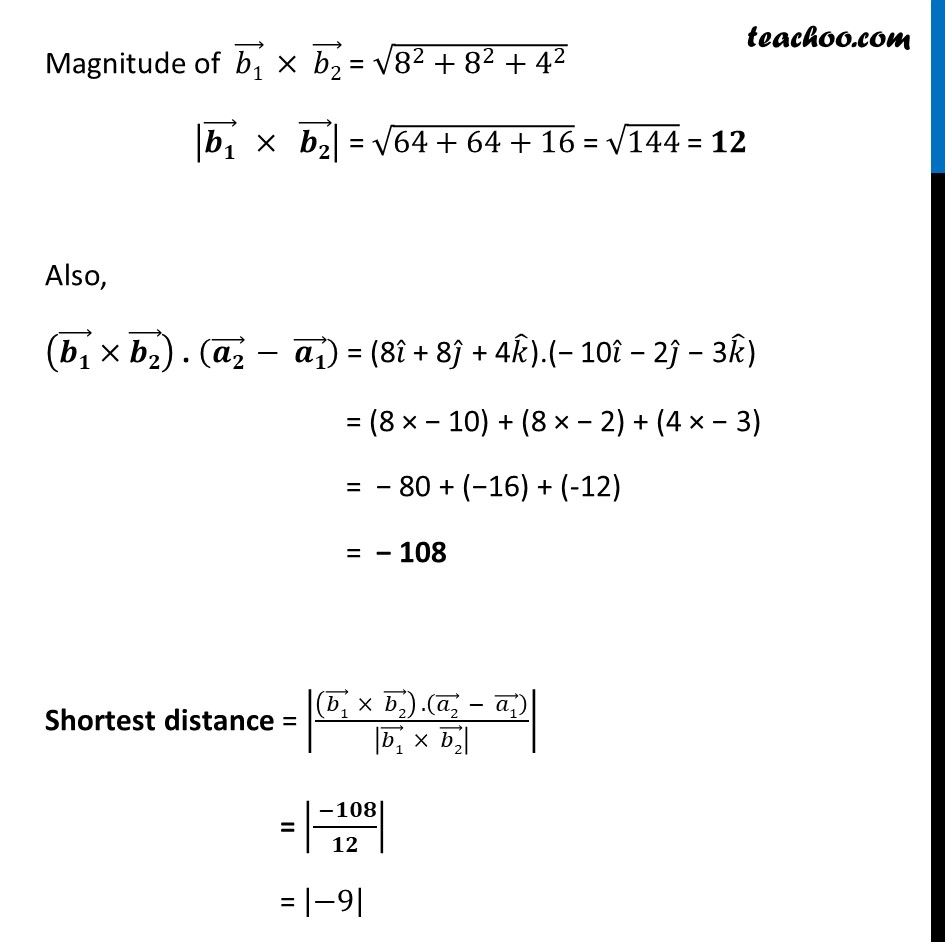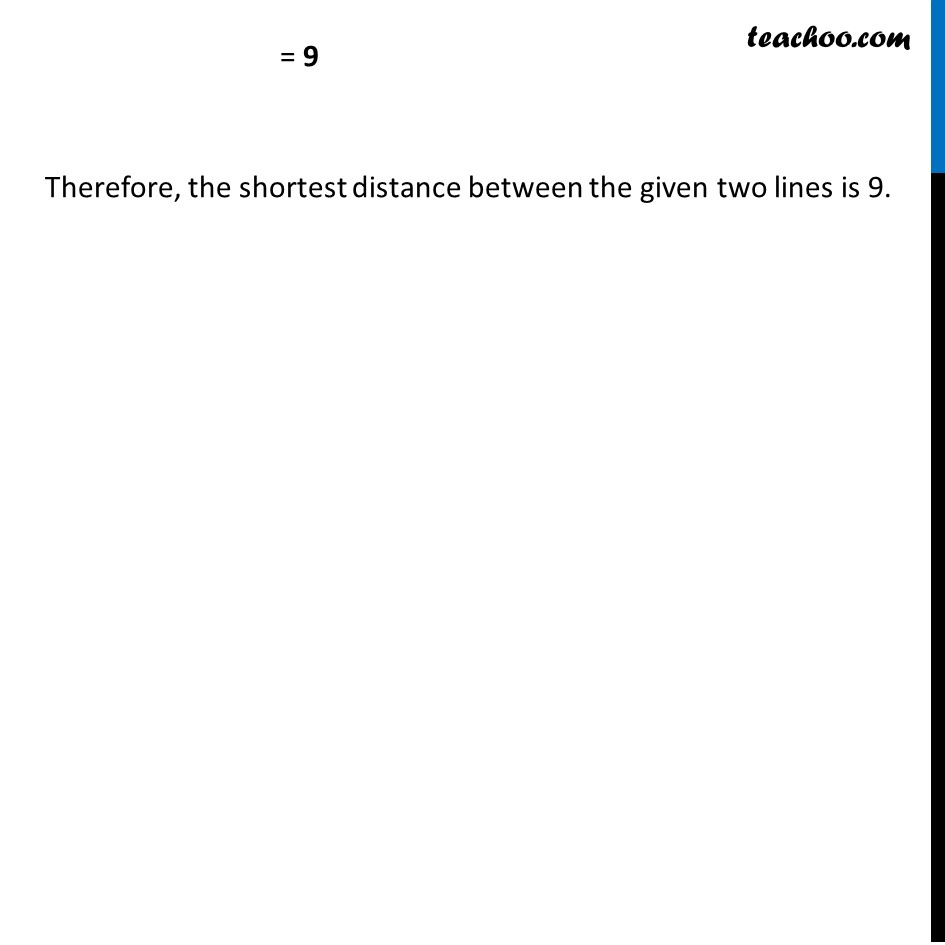Miscellaneous

Chapter 11 Class 12 Three Dimensional Geometry
Serial order wiseLearn in your speed, with individual attention - Teachoo Maths 1-on-1 Class

### Transcript

Misc 4 Find the shortest distance between lines 𝑟 ⃗ = 6𝑖 ̂ + 2𝑗 ̂ + 2𝑘 ̂ + 𝜆 (𝑖 ̂ – 2𝑗 ̂ + 2𝑘 ̂) and 𝑟 ⃗ = –4𝑖 ̂ – 𝑘 ̂ + 𝜇 (3𝑖 ̂ – 2𝑗 ̂ – 2𝑘 ̂) .Shortest distance between lines with vector equations 𝑟 ⃗ = (𝑎1) ⃗ + 𝜆 (𝑏1) ⃗ and 𝑟 ⃗ = (𝑎2) ⃗ + 𝜇(𝑏2) ⃗ is |(((𝒃𝟏) ⃗ × (𝒃𝟐) ⃗ ).((𝒂𝟐) ⃗ − (𝒂𝟏) ⃗ ))/|(𝒃𝟏) ⃗ × (𝒃𝟐) ⃗ | | 𝒓 ⃗ = (6𝒊 ̂ + 2𝒋 ̂ + 2𝒌 ̂) + 𝜆 (𝒊 ̂ − 2𝒋 ̂ + 2𝒌 ̂) Comparing with 𝑟 ⃗ = (𝑎1) ⃗ + 𝜆(𝑏1) ⃗ , (𝑎1) ⃗ = 6𝑖 ̂ + 2𝑗 ̂ + 2𝑘 ̂ & (𝑏1) ⃗ = 1𝑖 ̂ − 2𝑗 ̂ + 2𝑘 ̂ 𝒓 ⃗ = (−4𝒊 ̂ − 𝒌 ̂) + 𝝁 (3𝒊 ̂ − 2𝒋 ̂ − 2𝒌 ̂) Comparing with 𝑟 ⃗ = (𝑎2) ⃗ + 𝜇(𝑏2) ⃗ , (𝑎2) ⃗ = − 4𝑖 ̂ + 0𝑗 ̂ − 1𝑘 ̂ & (𝑏2) ⃗ = 3𝑖 ̂ − 2𝑗 ̂ − 2𝑘 ̂ Now, ((𝒂𝟐) ⃗ − (𝒂𝟏) ⃗) = (−4𝑖 ̂ + 0𝑗 ̂ − 1𝑘 ̂) − (6𝑖 ̂ + 2𝑗 ̂ + 2𝑘 ̂) = (−4 − 6) 𝑖 ̂ + (0 − 2)𝑗 ̂ + (−1 − 2) 𝑘 ̂ = − 10𝒊 ̂ − 2𝒋 ̂ − 3𝒌 ̂ ((𝒃𝟏) ⃗ × (𝒃𝟐) ⃗) = |■8(𝑖 ̂&𝑗 ̂&𝑘 ̂@1& −2&2@3&−2&−2)| = 𝑖 ̂ [(−2×−2)−(−2×2)] − 𝑗 ̂ [(1×−2)−(3×2)] + 𝑘 ̂ [(1×−2)−(3×−2)] = 𝑖 ̂ [ 4+4] − 𝑗 ̂ [−2−6] + 𝑘 ̂ [−2+6] = 𝑖 ̂ (8) − 𝑗 ̂ (−8) + 𝑘 ̂(4) = 8𝒊 ̂ + 8𝒋 ̂ + 4𝒌 ̂ Magnitude of (𝑏1) ⃗ × (𝑏2) ⃗ = √(8^2+8^2+4^2 ) |(𝒃𝟏) ⃗ × (𝒃𝟐) ⃗ | = √(64+64+16) = √144 = 𝟏𝟐 Also, ((𝒃𝟏) ⃗×(𝒃𝟐) ⃗ ) . ((𝒂𝟐) ⃗ − (𝒂𝟏) ⃗ ) = (8𝑖 ̂ + 8𝑗 ̂ + 4𝑘 ̂).(− 10𝑖 ̂ − 2𝑗 ̂ − 3𝑘 ̂) = (8 × − 10) + (8 × − 2) + (4 × − 3) = − 80 + (−16) + (-12) = − 108 Shortest distance = |(((𝑏1) ⃗ × (𝑏2) ⃗ ) . ((𝑎2) ⃗ − (𝑎1) ⃗ ))/|(𝑏1) ⃗ × (𝑏2) ⃗ | | = |( −𝟏𝟎𝟖)/𝟏𝟐| = |−9| = 9 Therefore, the shortest distance between the given two lines is 9.